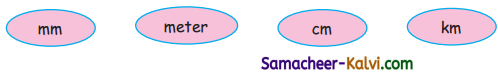## Samacheer Kalvi 3rd Standard Maths Book Solutions Term 1 Chapter 4 Measurements

Activity 1 (Text Book Page No. 45):

Tabulate the length of your class table measured by five of your friends with non-standard units.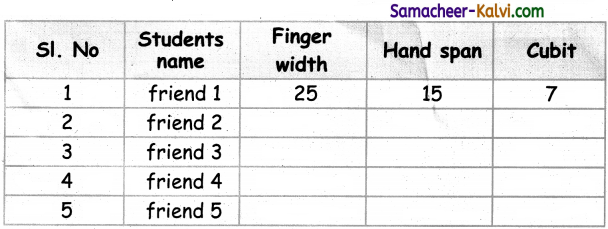Activity to be done by the students themselves.Activity 2 (Text Book Page No. 45):

Measure the length of ribbon by your hand span.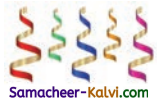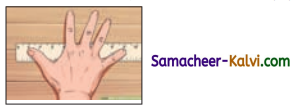Friend 1 ___________ hand span
Friend 2 ___________ hand span
Friend 3 ___________ hand span
Friend 4 ___________ hand span
Activity to be done by the students themselves.Activity 3 (Text Book Page No. 45):

Students are divided into two groups. One group should measure the length of the class room in non standard units and the other group in standard units.
Activity to be done by the students themselves.

Activity 4 (Text Book Page No. 45):

Group of five students measure the length of the black board by given objects.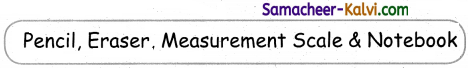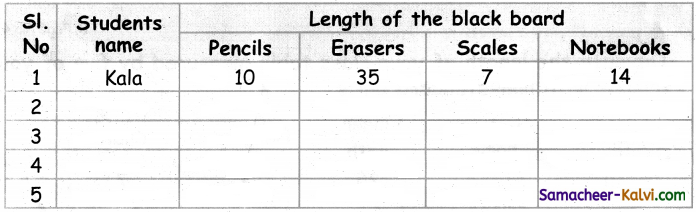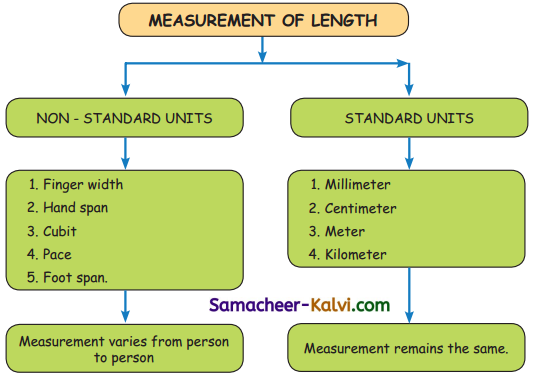Activity 5 (Text Book Page No. 47):

Measure the length of ribbon by tape roll.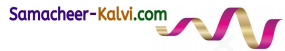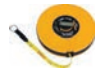Raj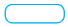centimeter
Kavicentimeter
Anucentimeter
Kavicentimeter

Measurements measured using standard units do not change from person to person.
Activity to be done by the students themselves.Practice (Text Book Page No. 47):

Question 1.
Measure the length of following things by centimetre scale and write it in the table below.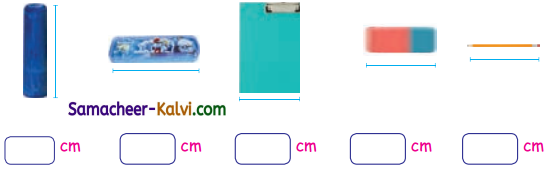Activity to be done by the students themselves.Question 2.
Measure length of the things by using ruler.

Measure the length of the following things in your house using a scale.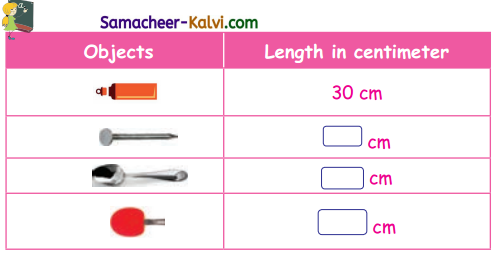Activity to be done by the students themselves.Try this (Text Book Page No. 48):

a. Write metre or centimetre in the blank space.

i. My pencil is 6 _____ long.
cm

ii. My hairpin is _____ long.
mm

iii. This tree is 3 _____ high.
m

iv. Height of coconut tree is 15 _____.
m

b. Meena has a ribbon of 50 cm and Reena has a ribbon of 110 cm. Whose ribbon is the longer?
Meena’s Ribbon = 50 cm
Reena’s Ribbon = 110 cm
∴ Reena’s Ribbon is longer.Activity 6 (Text Book Page No. 48):

Measure the length of following things in your class room using metre tape and fill in the boxes.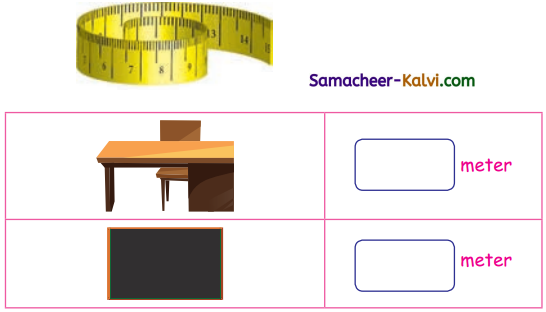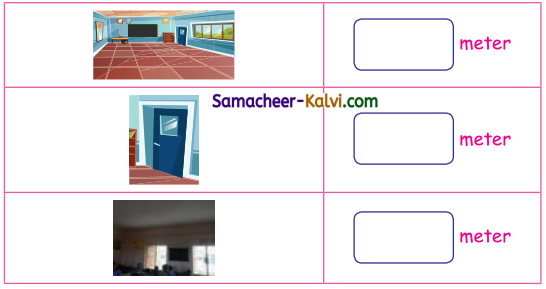Activity to be done by students.Try this (Text Book Page No. 48):

a. Centimeter _____ Meter
<

b. Meter _____ Kilometer
<

c. Kilometer _____ Centimeter
>

Practice (Text Book Page No. 49):

Match the objects with the appropriate units of measuring their length.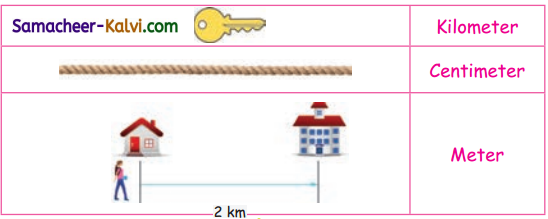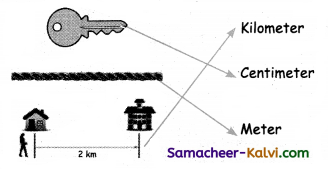Activity 7 (Text Book Page No. 49):

Estimate the length of the following objects and verify with actual length.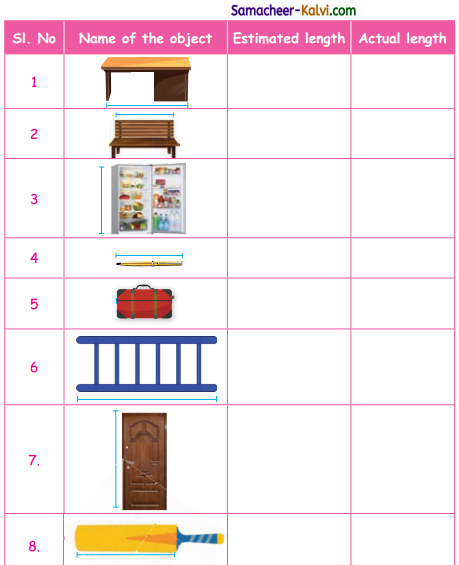Activity to be done by students.Practice (Text Book Page No. 50):

Question 1.
Circle the odd one.
1. mm
2. cm
3. m
4. cubit
4. cubit

Question 2.
Fill in the blanks.

a. 1 meter = _____ cms
100

b. 2 meter = _____ cms
200

c. 3 meter = _____ cms
300

d. 4 meter = _____ cms
400Question 3.
Match the following.

 10 millimeters 1 kilommeter 100 centimeters 1 centimeter 1000 meters 1 meter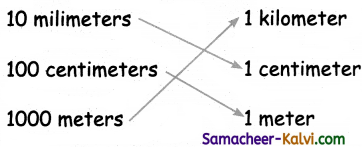Question 4.
Write all the non-standard units.

1. Finger span
2. Hand span
3. Cubit
4. Pace
5. Foot spanQuestion 5.
Write all the standard units you know.

1. Millimeter
2. Centimeter
3. Meter
4. Kilometer

Question 6.
Write in short form,

a. millimeter : _____
mm

b. meter : _____
mQuestion 7.
Write the given units in order.
Ascending order: _____, _____, _____, _____
Descending order: _____, _____, _____, _____